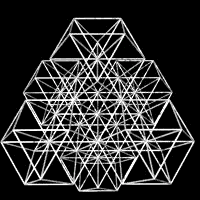# SPHERE PACKING STUDIES

Synergetics takes up the subject of spheres packed tightly together. Mathematicians have not yet reached consensus on a proof that a Barlow packing, including the face-centered cubic (fcc) and hexagonal (hcp) is actually the densest possible, although Gauss proved the fcc's density of approximately 0.74 optimal for a lattice (any denser arrangement would have to be more random).

The fcc packing is easily described in terms of adding successive layers of spheres to a tetrahedron. Canon balls and fruits in the grocery store are typically stacked in this fashion. A single orange nestles in the "valley" formed by three below it. A triangular layer of six oranges underlies that one and so on. Each layer adds a certain number of oranges, which may be expressed as a function of the growing tetrahedral shape's 'frequency'. The frequency is equivalent to the number of intervals between oranges along the tetrahedron's edge.

```        *         *       *      *
* *       * *     * *
* * *     * * *
* * * *

Fig. 1.  Tri-ville Packing (or Pool Ball)

```

Kepler studied sphere packing pretty intensely and knew that you get the same fcc packing if you start with a layer of spheres packed in a square arrangement and nest the next layer in the valleys so formed. If you taper off as you go upwards, this looks kind of like a Mayan Temple, so I call it "Mayan temple packing".

```
* * * *    * * *    * *     *
* * * *    * * *    * *
* * * *    * * *
* * * *

Fig. 2.  Squares-ville Packing (or Mayan Temple)

```

As Jim Morrissett pointed out to me during an IRC chat one morning, the Mayan temple packing forces the fcc, whereas the pool ball packing does not. This is because a "squares-ville" layer presents only one set of valleys for the next layer of spheres, whereas a "tri-ville" layer presents twice as many valleys as we will find usable -- presents an alternative, allowing us to go for hcp instead of fcc or some other Barlow packing.

The table below has a slightly different focus from Fuller's in Synergetics in Synergetics Principles ( section 220.00). Fuller's 2nd power derivations were of the form 2 p ff + 2 where 2 p is the number of non-polar vertices (V-2). This expresses the number of spheres in the outer layer of a shape as a function of frequency. Below, the focus is on the number of spheres added as a shape grows in size, versus the number of spheres exposed on a surface. For example, a tetrahedron starts with a nuclear sphere, and then an expanding base of 3, 6, 10... new spheres per layer.The octahedron and cuboctahedron are other shapes which grow through successive frequencies. For example, the cuboctahedron begins at Frequency zero with a single sphere. When twelve spheres close-pack around it, we get a one-frequency cuboctahedron. With frequency-2, we find 42 more spheres get added, then 92 and so on. Again, the number of spheres added per frequency is expressible in terms of a mathematical formula, as well as the cumulative total number of spheres in the growing cuboctahedron.Spheres packed in an icosahedral conformation have the same number of spheres as in a shell of the cuboctahedron of the same frequency, although not cumulatively. This fact has proved useful in virology, where many viruses have icosahedral encasements composed of capsomeres. The half-octahedron is like an Egyptian pyramid in shape. It's base consists of spheres arranged in a square n-by-n pattern. Each layer above the base is one sphere less along each edge. In other words, a five-layer half-octa consists of 25+9+4+1 spheres, or 39 total. Since the number of layers is one more than the frequency, our equations start with one for F=0. When F increases to one, four spheres are added, giving a total of five spheres etc. The octahedron is the same as a half-octa but with layers building from the base in both directions. Counting all the spheres in the half-octa twice, with the exception of those in the "middle" layer (formerly the base), gives us a way to derive an equation for the number of spheres added per frequency, along with a cumulative total equation. All of the packings described above are equivalent to the face-centered cubic. Connecting the centers of adjacent spheres with rods, and allowing the spheres to fade from view, is what gives us the isotropic vector matrix in synergetics.As Robert W. Gray pointed out in a posting to Synergetics-L, the 2nd column of formulae in the above chart derive easily from those in the first column, given that the sum of successive 2nd powers (1+4+9+...) is given by n(n+1)(2n+1)/6 (n=number of terms), which formula may be proved by mathematical induction. Jakob Bernoulli derived the general formula for the sum of successive n-powered terms in about 1690. See A Study in Sphere Packing for a more computational approach to sphere packing in a cuboctahedral conformation (IVM-style). Relevant readings:

Synergetics on the Web
maintained by Kirby Urner

12-around-1 graphic by Richard Hawkins [rh] using
Alias Animator V5.1 on an SGI Indigo2 Extreme
chardhawk@nets.com

Equations developed and depicted using MathCad 6.0

Thanks to Kevin Brown for email re Bernoulli, and to Dr. John Conway
for a lot of historical information and up-to-date nomenclature.